# GSEB Solutions Class 12 Maths Chapter 8 Application of Integrals Miscellaneous Exercise

Gujarat Board GSEB Textbook Solutions Class 12 Maths Chapter 8 Application of Integrals Miscellaneous Exercise Textbook Questions and Answers.

## Gujarat Board Textbook Solutions Class 12 Maths Chapter 8 Application of Integrals Miscellaneous Exercise

Question 1.
Find the area under the following given curves and given lines:
(i) y = x2, x = 1, x = 2 and x-axis.
(ii) y = x4, x = 1, x = 5 and x-axis.
Solution:
(i) Graphs of parabola y = x2, lines x = 1, x = 2 and x-axis are shown in the figure.
The area of region bounded by y = x2, x = 1, x = 2 and x-axis.
= Area of region PLMQP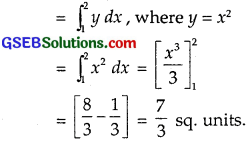(ii) The curve y = x4 passes through (0, 0) and it is symmetric about y-axis for all x ∈ R.∴ $$\frac{dy}{dx}$$ = 4x3.
$$\frac{dy}{dx}$$ = 0 at x = 0.
$$\frac{dy}{dx}$$ changes sign from – ve to + ve as x increases through x = 0
∴ y is minimum at x = 0.
When x > 0, y is the increasing function.
Graph is as shown in the figure.
Area of region bounded by
y = x4, x = 1,
x = 5 and x-axis
= Area of region ABQPA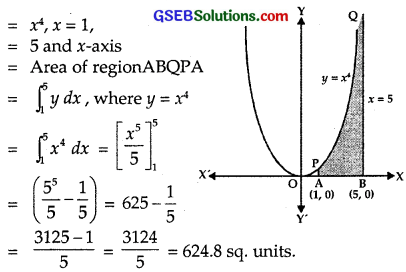Question 2.
Find the area between the curves y = x and y = x2.
Solution:
Given curves are
y = x …………… (1)
y = x2 ……………… (2)
Putting y = x in eq.(2), we get
x = x2
∴ x = 0, 1
When x = 1, y = 1These curves intersect at (0, 0) and (1, 1).
∴ Area between y = x
and y = x2
= Area of the region OCPQ
= Area of OAP – Area of region OAPCO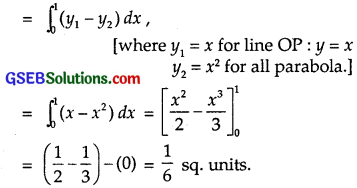Question 3.
Find the area of the region lying in first quadrant and bounded by y = 4x2, x = 0, y = 1 and y = 4.
Solution:
The given curve and lines which bound the region are
y = 4x2, x = 0, y = 1 and y = 4.
Graph is as shown in the figure.
The area of the region lying in the first quadrant and bounded by
y = 4x2, x = 0, y = 1 and y = 4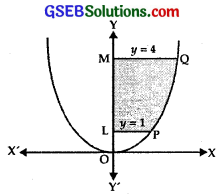= Area of the region = LPQML
= Area OQMO – Area OPLOQuestion 4.
Sketch the graph of y = |x + 3| and evaluate $$\int_{6}^{0}$$ |x + 3| dx.
Solution:
y = |x + 3|
At x = – 3, y = 0
AQ is the line y = x + 3.
When x + 3 < 0,
y = -(x + 3)
= – x – 3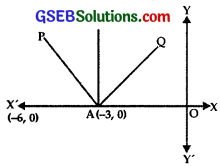Graph of the line is AP.
∴ Graph of y = |x + 3| is as shown in the figure.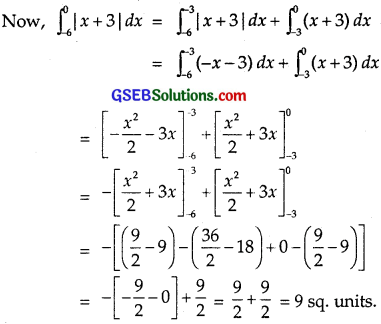Question 5.
Find the area bounded by the curve y = sin x between x = 0 and x = 2π.
Solution:
Some points on the sine graph are:Plotting these points, we get the graph OPAQB.
Since sin(2π – x) = – sin x, therefore
graph between x = π and x = 2π has the same shape but it is below the x-axis.
Area of the region OPA = Area of the region AQB.
∴ Area bounded by the curve y = sin x between x = 0 and x = 2π.
= 2 × Area of the region OPA= – 2[cos π – cos 0] = 2[1 + 1] = 4.

Question 6.
Find the area enclosed between the parabola y2 = 4ax and the line y = mx.
Solution:
The given curves are
y = mx …………….. (1)
y2 = 4ax …………….. (2)
Putting value of y from (1) in (2), we get
m2x2 = 4ax
or x(m2x – 4a) = 0
∴ x = 0, x = $$\frac{4 a}{m^{2}}$$.
Putting x = $$\frac{4 a}{m^{2}}$$ in (1),
y = m.$$\frac{4 a}{m^{2}}$$ = $$\frac{4a}{m}$$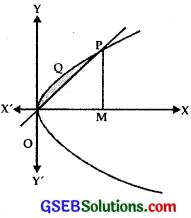∴ The curve y2 = 4ax and OP intersect at O(0, 0) and
∴ Area enclosed between the parabola y2 = 4ax and the line y = mx.
= Area of the region OPOQ [As per figure]
= Area of the region OMPQO – Area of ∆ OMP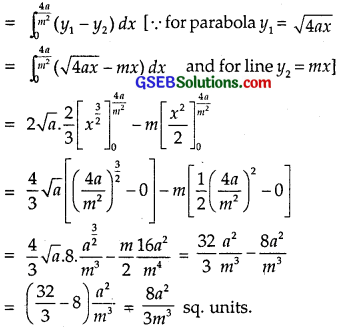Question 7.
Find the area enclosed by the parabola 4y = 3x2 and the line 2y = 3x + 12.
Solution:
The parabola and the line are
4y = 3x2 …………………. (1)
2y = 3sx + 12 ………………. (2)
Multiplying (2) by 2 and
Subtracting from (1), we get
0 = 3x2 – 6x – 24
or x2 – 2x – 8 = 0
or (x – 4)(x + 2) = 0
∴ x = 4, – 2
From (2), y = 12, 3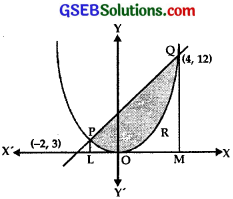The graph of parabola and lines are shown in the figure. They intersect at P(- 2, 3) and Q(4, 12).
∴ The area enclosed by the parabola 4y = 3x2
and the line 2y = 3x + 12
= Area of the region POPQP
= Area of trapezium PLMQP – Area of the region LMQROP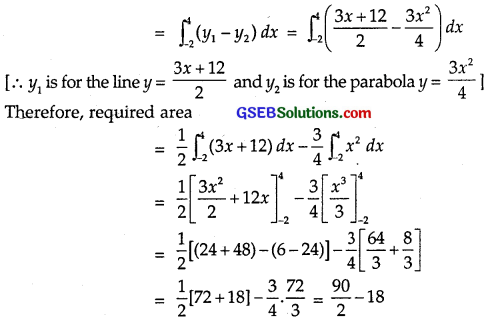= 45 – 18 = 27 sq.units.

Question 8.
Find the area of the smaller region bounded by the ellipse
$$\frac{x^{2}}{9}$$ + $$\frac{y^{2}}{4}$$ = 1 and the straight line $$\frac{x}{3}$$ + $$\frac{y}{2}$$ = 1.
Solution:It is an ellipse with vertices at A(3, 0) and B(0, 2) and length of the major axis = 2(3) = 6 and length of the minor axis 2(2) = 4.
Line $$\frac{x}{3}$$ + $$\frac{y}{2}$$ = 1 ⇒ y = ($$\frac{6-2x}{3}$$)
It is a straight line passing through A(3, 0) and B(0, 2).
Smaller area common to both is shaded.For I1 put x = 3 sin θ so that dx = 3 cos θ dθ.
When x = 0, θ = 0 and when x = 3, θ = $$\frac{π}{2}$$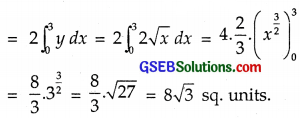Question 9.
Find the area of the smaller region bounded by the ellipse
$$\frac{x^{2}}{a^{2}}$$ + $$\frac{y^{2}}{b^{2}}$$ = 1 and the staraight line $$\frac{x}{a}$$ + $$\frac{y}{b}$$ = 1.
Solution:Question 10.
Find the area of the region enclosed by parabola x2 = y, the line y = x + 2 and the x-axis.
Solution:
We have x2 = y. It represents a parabola with vertex at (0, 0), axis along the positive direction of y-axis and it opens upwards.
Also, y = x + 2 represents a straight line cutting x-axis at (-2, 0).
Solving x2 = y and y = x + 2, we get
x2 = x + 2
⇒ x2 – x – 2 = 0
⇒ (x – 2)(x + 1) = 0
⇒ x = 2, x = 1.
⇒ When x = 2, y = (2)2 = 4
and when x = – 1, y = (- 1)2 = 1.So the two curves x2 = y and y = x + 2 intersect at the points (2, 4) and (- 1, 1).
Required area = Shaded region shown in the figureQuestion 11.
Using method of integration, find the area bounded by |x| + |y| = 1.
Solution:
In I Quadrant, x > 0 and y > 0.
⇒ |x| = x, |y| = y.
The line is x + y = 1 …………….. (1)
In II Quadrant, x < 0 and y > 0.
⇒ |x| = – x. |y| = y.
The line is – x + y = 1
or x – y = -1 ………………… (2)
In III Quadrant, x < 0 and y < 0. ⇒ |x| = – x, |y| = – y. The line is – x – y = 1 or x + y = -1 ………….. (3)
In IV Quadrant, x > 0 and y < 0.
⇒ |x| = x, |y| = – y,
The line is x – y = 1 …………. (4)
Thus, |x| + |y| = 1 represent four lines forming a square ABCD.
Area of square ABCD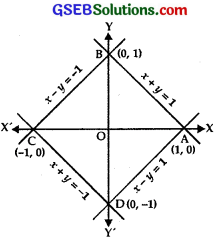= 4 × Area of ∆ AOB
= 4 × $$\int_{0}^{1}$$ (1 – x) dx
Since x + y = 1 is the equation of the line AB.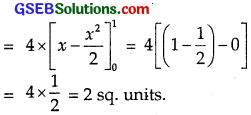= 4 × $$\frac{1}{2}$$ = 2 sq.units.

Question 12.
Find the area bounded by the curves {(x, y) y = ≥ x2 and y = |x|}.
Solution:
Clearly, x2 = y represents parabola with vertex at (0, 0), positive direction of y-axis as its axis and it opens upwards.
y= | x |, i.e., y = x and y = – x represent two lines passing through the origin and making an angle of 45° and 135° with the positive direction of the x-axis.The required region is the shaded region as shown in the figure. Since both the curves are symmetrical about y-axis, therefore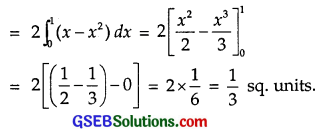Question 13.
Using the method of integration, find the area of the triangle ABC, coordinates of whose vertices are A(2, 0), B(4, 5) and C(6, 3).
Solution:
Equation of the line AB is
y – 0 = $$\frac{5-0}{4-2}$$(x – 2)
⇒ y = $$\frac{5}{2}$$(x – 2).
Equation of the line BC is
y – 5 = $$\frac{3-5}{6-4}$$(x – 4)
⇒ y = – x + 9.
Equation of the line CA is
y – 3 = $$\frac{0-3}{2-6}$$(x – 6)
⇒ y = $$\frac{3}{4}$$(x – 2).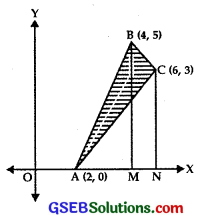Required area = area of the region bounded by ∆ ABC
= area of the region AMB + area of the region BMNC – area of the region ANC= 5 + 8 – 6 = 7 sq. units.

Question 14.
Using the method of integration, find the area of the region bounded by the lines
2x + y = 4, 3x – 2y = 6 and x – 3y + 5 = 0.
Solution:
The given lines are 2x + y = 4 ………….. (1)
3x – 2y = 6 ……………….. (2)
x – 3y = – 5 ……………….. (3)
Multiplying (3) by 2 and subtracting from (1), we get
7y = 14 ⇒ y = 2.
So, from (3) x – 6 = 5 ⇒ x = 1.
∴ Lines (1) and (3) intersect at (1, 2).
Again multiplying (3) by 3 and subtract it from (2). We get
7y = 21 ∴ y – 3.
From (3), X – 9 = – 5 ⇒ x = 4.
∴ Lines (2) and (3) intersect at (4, 3).
Multiply eq. (1) by 2 and add it to (2).
We get: 7x = 14 ∴ x = 2
From (2), 4 + y – 4 ∴ y = 0.
Lines (1) and (2) intersect at (2, 0).
The points A(1, 2), B(4, 3) and C(2, 3) are plotted and joined obtaining triangle ABC.Area of ∆ ABC = Area of trapezium ALMB – Area of ∆ ALC – Area of ∆ BCMQuestion 15.
Find the area of the region {(x, y) : y2 ≤ 4x, 4x2 + 4y2 ≤ 9}.
Solution:
y2 = 4x is a parabola whose vertex is the origin and 4x2 + 4y2 = 9
represents a circle whose centre is (0, 0) and radius = $$\frac{3}{2}$$.
On solving y2 = 4x
and x2 + y2 = $$\frac{9}{4}$$.
The points of intersection are P($$\frac{1}{2}$$, $$\sqrt{2}$$) and Q($$\frac{1}{2}$$, – $$\sqrt{2}$$).
Both the curves are symmetrical about x-axisRequired area = area of the shaded region
= 2(area of the region OAPO)
= 2[(area of the region OMPO) + (area of the region MAPM)]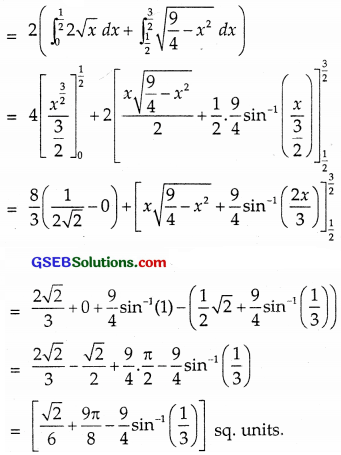Choose the correct answers in the following questions 16 to 19:

Question 16.
The area bounded by the curve y = x3, the x-axis and ordinates x = – 2 and x = 1 is
(A) – 9
(B) – $$\frac{15}{4}$$
(C) $$\frac{15}{4}$$
(D) $$\frac{17}{4}$$
Solution:
The curve is y = x3.
Differentiating, we get
$$\frac{dy}{dx}$$ = 3x2 = +ve∴ Curve is an increasing curve.
$$\frac{dy}{dx}$$ = 0 ⇒ x = 0.
∴ x-axis is the tangent at x = 0.
f(- x) = – f(x) ∴ (- x)3 = – x3.
Curve is symmetrical in opposite quadrants,
Area bounded by the curve y = x3, the x-axis, x = – 2 and x = 1
= Area of the region AQOBPOA
= Area of the region AQOA + Area of the region ∆ BPO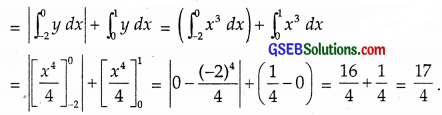∴ Part D is the correct answer.Question 17.
The area bounded by the curve y = x |x|, x-axis and the ordinates x = – 1 and x = 1 given by
(A) 0
(B) $$\frac{1}{3}$$
(C) $$\frac{2}{3}$$
(D) $$\frac{4}{3}$$
Solution:
When x > 0, |x| = x.
∴ The equation of the curve is y = x2.
When x < 0, |x| = – x.
∴ Equation of the curve is
y = x2.
∴ Area bounded by the curve
y = x|x|,
x-axis and ordinates x = – 1, x = 1
= Area of region + Area of region ∆ BQO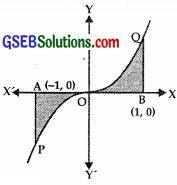= 2 × Area of region ∆ BQO
(∵ These areas are equal due to symmetry)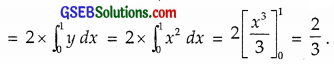∴ Part (C) is the correct answer.

Question 18.
The area of the circle x2 + y2 = 16 exterior to the parabola y2 = 6x is
(A) $$\frac{4}{3}$$ (4π – $$\sqrt{3}$$)
(B) $$\frac{4}{3}$$ (4π + $$\sqrt{3}$$)
(C) $$\frac{4}{3}$$ (8π – $$\sqrt{3}$$)
(D) $$\frac{4}{3}$$ (8π + $$\sqrt{3}$$)
Solution:
The given curves are
x2 + y2 = 16 ………….. (1)
y2 = 6x ……………….. (2)
Putting y2 = 6x in (1), we get
x2 + 6x = 16
or x2 + 6x – 16 = 0
(x + 8)(x – 2) = 0
∴ x = – 8, 2
But x ≠ – 8
So, x = 2.
From (2), y2 = 6x ⇒ y = ± 2$$\sqrt{3}$$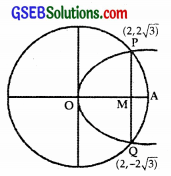Area of the whole circle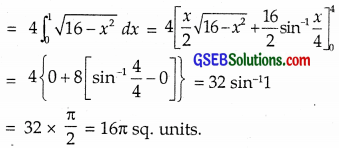Now circle and parabola intersect at P(2, 2$$\sqrt{3}$$) and Q (2, – 2$$\sqrt{3}$$).
Smaller area enclosed by circle and parabola
= Area of region OQAP
= 2 × Area of region OQAP
= 2 × [Area of region OMP + Area of region MAP]
= 2($$\int_{0}^{2} y_{1} d x+\int_{2}^{4} y_{2} d x$$)
When y1 is for parabola y2 = 6x. ∴ y = $$\sqrt{6x}$$.
y2 is for circle x2 + y2 = 16 ∴ y = $$\sqrt{16-x^{2}}$$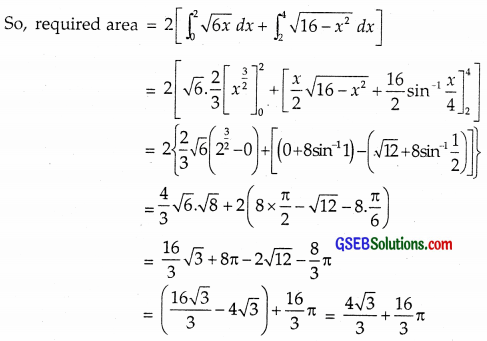Common area exterior to the parabola y2 = 6x is equal to
16π – ($$\frac{4 \sqrt{3}}{3}$$ + $$\frac{16}{3}$$π)
= $$\frac{32}{3}$$π – $$\frac{4 \sqrt{3}}{3}$$ = $$\frac{4}{3}$$(8π – $$\sqrt{3}$$).
∴ Part (C) is the correct answer.Question 19.
The area bounded by y-axis, y = cos x and y = sin x, 0 ≤ x ≤ $$\frac{π}{2}$$ is
(A) 2($$\sqrt{2}$$ – 1)
(B) $$\sqrt{2}$$ – 1
(C) $$\sqrt{2}$$ + 1
(D) $$\sqrt{2}$$
Solution:
The curve are y = cos x, y = sin x, 0 ≤ x ≤ $$\frac{π}{2}$$.
The curve meet
where sin x = cos x.
or tan x = 1
⇒ x = $$\frac{π}{4}$$.
sin $$\frac{π}{4}$$ = cos $$\frac{π}{4}$$ = $$\frac{1}{\sqrt{2}}$$.Graphs of these curves are as shown in the figure.
They intersect at P($$\frac{π}{4}$$, $$\frac{1}{\sqrt{2}}$$).
The area bounded by y-axis, y = cos x and y = sin x (0 ≤ x ≤ $$\frac{π}{2}$$)